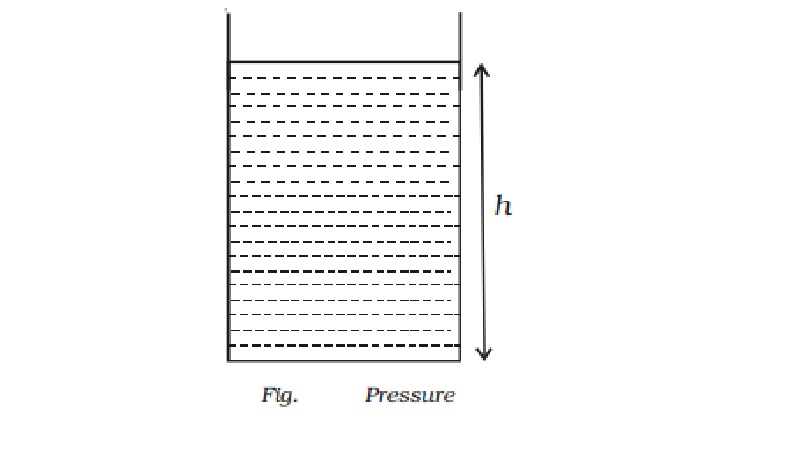Home | | Physics | | Physics | Pressure due to a liquid column

# Pressure due to a liquid columnA fluid is a substance that can flow when external force is applied on it. The term fluids include both liquids and gases. Though liquids and gases are termed as fluids, there are marked differences between them.

Fluids

A fluid is a substance that can flow when external force is applied on it. The term fluids include both liquids and gases. Though liquids and gases are termed as fluids, there are marked differences between them. For example, gases are compressible whereas liquids are nearly incompressible. We only use those properties of liquids and gases, which are linked with their ability to flow, while discussing the mechanics of fluids.Pressure due to a liquid column

Let h be the height of the liquid column in a cylinder of cross sectional area A. If ρ is the density of the liquid, then weight of the liquid column W is given by

W = mass of liquid column � g = Ahρg

By definition, pressure is the force acting per unit area

Pressure =  weight of liquid column / area of cross section

= Ah g / A

= hρg

Pascal's law

One of the most important facts about fluid pressure is that a change in pressure at one part of the liquid will be transmitted without any change to other parts. This was put forward by Blaise Pascal (1623 - 1662), a French mathematician and physicist. This rule is known as Pascal's law.

Pascal's law states that if the effect of gravity can be neglected then the pressure in a fluid in equilibrium is the same everywhere.

Study Material, Lecturing Notes, Assignment, Reference, Wiki description explanation, brief detail

Related Topics